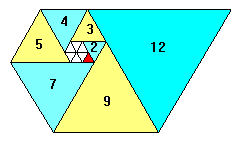# Number Theory: Notes by Anwar Khan

These notes are in two part. One is “number theroy” and other one is “algebraric number theroy”. These notes are provided by Mr. Anwar Khan. We are very thankful to him for providing these notes.Name Number Theory: Notes Anwar Khan 219 pages PDF 4.5 mB
• Number Theory
• Divisibility
• Euclid’s Theorem
• Common Division
• Method of finding great common divisor
• G.C.D. more than two integers
• Least Common Multiple
• The Linear Diophantine Equation
• Theory of Primes
• Composite Number
• Prime Divisor
• Congruence
• Complete Residue System
• Solutions of the Congruence
• Perfect Number
• Reduce residue System
• Arithmetic Function

# Algebraic Number Theory: Notes by Anwar Khan

 Name Algebraic Number Theory: Notes Anwar Khan 110 pages PDF 2.8 mB
• Algebraic Number Theory \dotfill 1
• Diophantine Equation and Fermat’s Conjecture \dotfill 2
• Polynomial over the Rational \dotfill 10
• Degree of Polynomial \dotfill 10
• Monic Polynomial
• Division of Polynomial
• Irreducible Polynomial
• Division Algorithm
• Greatest Common Divisor
• Algebraic Number
• Degree of Algebraic Number
• Minimal Polynomial
• Conjugates of an Algebraic Number
• Primitive Polynomial
• Product of Polynomial
• Symmetric Polynomial
• Alternative Statement
• Primitive Element
• Eisenstein’s Irreducibility Eriterion
• Algebraic Integer
• The Determinant
• Euclidian Domain
• Square Free Rational Integer
• Prime
• Ideal
• Principal Ideal
• Congruence of an Ideal
• Norm f an Ideal
• Decrement
• Units and Primes in $\mathbb{R}$
• Unique Factorization Domain
• Arithmetic of an Ideal
• Prime Ideal
• Equivalent Ideal
• Cyclotomic Field KP
• Pure Cubic Field
• msc/notes/number_theory_by_anwar_khan